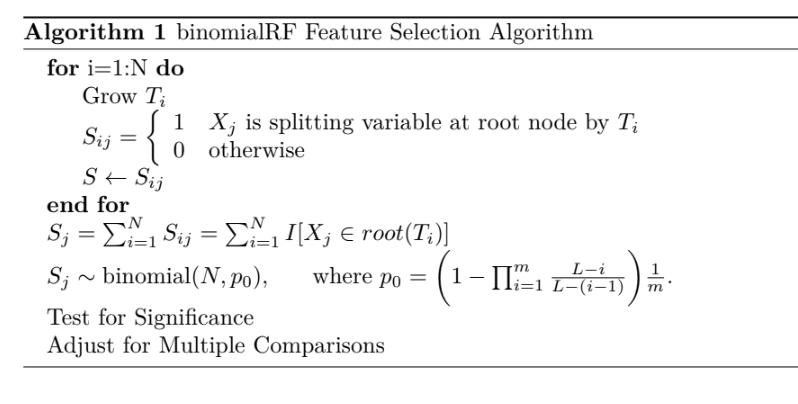# binomialRF Feature Selection Vignette

#### 2020-03-26

The $$\textit{binomialRF}$$ is a $$\textit{randomForest}$$ feature selection wrapper (Zaim 2019) that treats the random forest as a binomial process where each tree represents an iid bernoulli random variable for the event of selecting $$X_j$$ as the main splitting variable at a given tree. The algorithm below describes the technical aspects of the algorithm.binomialRF Algorithm.

# Simulating Data

Since $$\textit{binomialRF}$$ is a wrapper algorithm that internally calls and grows a randomForest object based on the inputted parameters. First we generate a simple simulated logistic data as follows:

• $$X_{10}\sim MNV(0, I_{10})$$,

• $$p(x) = \frac{1}{1+e^{-X\beta}}$$, and

• $$y \sim Binom(10,p)$$.

where $$\beta$$ is a vector of coefficients where the first 2 coefficients are set to 3, and the rest are 0.

$\beta = \begin{bmatrix} 3 & 3 & 0 & \cdots & 0 \end{bmatrix}^T$

## Simulated Data

set.seed(324)

### Generate multivariate normal data in R10
X = matrix(rnorm(1000), ncol=10)

### let half of the coefficients be 0, the other be 10
trueBeta= c(rep(3,2), rep(0,8))

### do logistic transform and generate the labels
z = 1 + X %*% trueBeta
pr = 1/(1+exp(-z))
y = rbinom(100,1,pr)

To generate data looking like this:

y
0.61 1.13 -0.30 0.11 0.20 1.11 1.51 -0.44 -0.39 -1.87 1
0.19 0.13 -0.99 -0.41 -0.49 1.07 2.33 0.72 0.34 0.97 1
0.54 -1.00 -0.47 -0.48 1.74 0.23 0.13 0.95 -0.99 0.12 1
0.56 -2.52 0.82 0.44 1.24 -0.01 0.11 -0.51 0.39 1.24 0
-0.64 -1.63 1.93 -0.71 -0.68 0.13 -0.01 0.66 -0.23 0.38 0
1.22 -1.06 -0.06 0.09 1.59 1.39 -1.78 -0.92 -0.16 0.00 1
1.27 -0.81 1.18 0.23 0.90 0.35 0.58 -0.83 0.25 1.79 1
-0.57 1.51 0.39 -1.74 -0.57 -0.40 1.12 0.76 0.44 1.11 1
-0.62 -0.92 -1.19 0.23 -0.05 -1.18 -0.25 -1.73 -1.27 -0.04 0
-0.97 0.43 -1.13 -0.18 -0.59 -1.76 -0.62 0.72 0.12 0.73 0

## Generating the Stable Correlated Binomial Distribution

Since the binomialRF requires a correlation adjustment to adjust for the tree-to-tree sampling correlation, we first generate the appropriately-parameterized stable correlated binomial distribution. Note, the correlbinom function call can take a while to execute for large number trials (i.e., trials > 1000).

require(correlbinom)
rho = 0.33
ntrees = 250

cbinom = correlbinom(rho, successprob =  1/ncol(X), trials = ntrees,
precision = 1024, model = 'kuk')

## binomialRF Function Call

Then we can run the binomialRF function call as below:

binom.rf <- binomialRF::binomialRF(X,factor(y), fdr.threshold = .05,
ntrees = ntrees,percent_features = .6,
fdr.method = 'BY', user_cbinom_dist = cbinom,
sampsize = round(nrow(X)*.33))
print(binom.rf)
#>     variable freq significance adjSignificance
#> X2        X2  114 0.000000e+00     0.00000e+00
#> X1        X1   94 3.765189e-09     4.79322e-08
#> X3        X3   23 9.999999e-01     1.00000e+00
#> X6        X6    6 1.000000e+00     1.00000e+00
#> X7        X7    5 1.000000e+00     1.00000e+00
#> X8        X8    5 1.000000e+00     1.00000e+00
#> X4        X4    1 1.000000e+00     1.00000e+00
#> X5        X5    1 1.000000e+00     1.00000e+00
#> X10      X10    1 1.000000e+00     1.00000e+00

# Tuning Parameters

## Percent_features

Note that since the binomial exact test is contingent on a test statistic measuring the likelihood of selecting a feature, if there is a dominant feature, then it will render all remaining ‘important’ features useless as it will always be selected as the splitting variable. So it is important to set the $$percent_features$$ parameter < 1. The results below show how setting the parameter to a fraction between .6 to 1 can allow other features to stand out as important.

#>
#>
#> binomialRF 100%
#>    variable freq significance adjSignificance
#> X2       X2  151 0.000000e+00    0.000000e+00
#> X1       X1   88 3.616441e-07    2.658084e-06
#> X3       X3    8 1.000000e+00    1.000000e+00
#> X5       X5    1 1.000000e+00    1.000000e+00
#> X6       X6    1 1.000000e+00    1.000000e+00
#> X8       X8    1 1.000000e+00    1.000000e+00
#>
#>
#> binomialRF 80%
#>     variable freq significance adjSignificance
#> X2        X2  128 0.000000e+00     0.000000000
#> X1        X1   83 1.021044e-05     0.000111002
#> X3        X3   25 9.999994e-01     1.000000000
#> X6        X6    5 1.000000e+00     1.000000000
#> X7        X7    4 1.000000e+00     1.000000000
#> X8        X8    2 1.000000e+00     1.000000000
#> X10      X10    2 1.000000e+00     1.000000000
#> X4        X4    1 1.000000e+00     1.000000000
#>
#>
#> binomialRF 60%
#>     variable freq significance adjSignificance
#> X2        X2  114 0.000000e+00    0.000000e+00
#> X1        X1   84 5.416284e-06    7.932062e-05
#> X3        X3   20 1.000000e+00    1.000000e+00
#> X6        X6    8 1.000000e+00    1.000000e+00
#> X4        X4    6 1.000000e+00    1.000000e+00
#> X7        X7    4 1.000000e+00    1.000000e+00
#> X8        X8    4 1.000000e+00    1.000000e+00
#> X9        X9    4 1.000000e+00    1.000000e+00
#> X5        X5    3 1.000000e+00    1.000000e+00
#> X10      X10    3 1.000000e+00    1.000000e+00

## ntrees

We recommend growing at least 500 to 1,000 trees at a minimum so that the algorithm has a chance to stabilize, but also recommend choosing ntrees as a function of the number of features in your dataset. The ntrees tuning parameter must be set in conjunction with the percent_features as these two are inter-connectedm as well as the number of true features in the model. Since the correlbinom function call is slow to execute for ntrees > 1000, we recommend growing random forests with only 500-1000 trees.

#>
#>
#> binomialRF 250 trees
#>     variable freq significance adjSignificance
#> X2        X2  191 0.000000e+00     0.00000e+00
#> X1        X1  172 1.406475e-11     2.05976e-10
#> X3        X3   64 9.999997e-01     1.00000e+00
#> X7        X7   26 1.000000e+00     1.00000e+00
#> X6        X6    9 1.000000e+00     1.00000e+00
#> X10      X10    9 1.000000e+00     1.00000e+00
#> X4        X4    8 1.000000e+00     1.00000e+00
#> X5        X5    8 1.000000e+00     1.00000e+00
#> X9        X9    8 1.000000e+00     1.00000e+00
#> X8        X8    5 1.000000e+00     1.00000e+00
#>
#>
#> binomialRF 500 trees
#>     variable freq significance adjSignificance
#> X2        X2   91 3.978159e-08    1.165190e-06
#> X1        X1   87 7.297797e-07    1.068751e-05
#> X3        X3   35 9.988915e-01    1.000000e+00
#> X6        X6   12 1.000000e+00    1.000000e+00
#> X7        X7   11 1.000000e+00    1.000000e+00
#> X8        X8    5 1.000000e+00    1.000000e+00
#> X9        X9    3 1.000000e+00    1.000000e+00
#> X10      X10    3 1.000000e+00    1.000000e+00
#> X5        X5    2 1.000000e+00    1.000000e+00
#> X4        X4    1 1.000000e+00    1.000000e+00